# Using The FAµST Projectors API

This notebook put the focus on the proj module of the pyfaust API. This module provides a bunch of projectors which are for instance necessary to implement proximal operators in PALM algorithms.
Indeed these projectors matter in the parametrization of the PALM4MSA and hierarchical factorization algorithms, so let's maintain their configuration as simple as possible by using projectors!
First let's explain some generalities about projectors:
• They are all functor objects (objects that you can call as a function).
• They are all types defined by child classes of the parent abstract class proj_gen.
The general pattern to use a projector unfolds in two steps:
1. Instantiate the projector passing the proper arguments.
2. Call this projector (again, as a function) on the matrix you're working on. This step is optional or for test purposes, because generally it is the algorithm implementation responsibility to call the projectors. You just need to feed the algorithms (PALM4MSA for example) with them.
Let's see how to define and use the projectors in the code. For the brief math definitions, I let you consult this document. Remember that the projector API is documented too, you'll find the link for each projector below. Last, if you're looking for a reference about proximal operators here it is: proximity operators.
The SP projector (projection onto matrices with a prescribed sparsity)
This projector performs a projection onto matrices with a prescribed sparsity. It governs the global sparsity of a matrix given an integer k. The matrix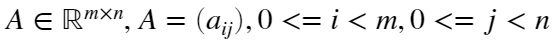, is projected to the closest matrix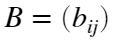such that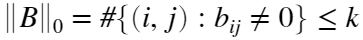which implies , if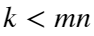, that some entries of A are kept in B and others are set to zero. The projector keeps the k most significant values (in term of absolute values or magnitude).
Let's try on an example, here a random matrix.
A = rand(5,5)*100
A = 5×5
35.7832 3.5555 73.1184 48.0426 95.1208 94.0956 22.3264 63.7686 90.9834 58.2946 44.4792 13.7963 97.5245 57.2125 39.1435 11.0819 30.2575 33.1082 42.4836 55.8078 86.5122 25.4192 42.0691 45.4418 98.6554
import matfaust.proj.sp
% 1. instantiate the projector
k = 2;
p = sp(size(A), k, 'normalized', false);
% 2. project the matrix through it
B = p(A)
B = 5×5
0 0 0 0 0 0 0 0 0 0 0 0 97.5245 0 0 0 0 0 0 0 0 0 0 0 98.6554
The projector is simply defined by the input matrix shape and the integer k to specify the targeted sparsity.
Optional normalization:
As you noticed, the argument normalized is set to false in the projector definition. This is the default behaviour. When normalized is true, the result B is normalized according to its Frobenius norm.
The next example gives you a concrete view of what happens when normalized is true.
pnorm = sp(size(A), k, 'normalized', true);
C = pnorm(A)
C = 5×5
0 0 0 0 0 0 0 0 0 0 0 0 0.7030 0 0 0 0 0 0 0 0 0 0 0 0.7112
disp( [ 'B/norm(B, "fro") == C: ', (int2str(norm(B/norm(B,'fro')-C) < 10^-6))])
B/norm(B, "fro") == C: 1
Sparsity and optional positivity:
It is also possible to "filter" the negative entries of A by setting the pos argument of sp to True. You can see the projector as a pipeline, the first stage is to filter out the negative values, then the sp projector is applied and finally the resulting image is normalized if normalized==True. The following example shows how the projector operates depending on combinations of pos and normalized values.
p_pos = sp(size(A), k, 'normalized', false, 'pos', true);
p_pos(A)
ans = 5×5
0 0 0 0 0 0 0 0 0 0 0 0 97.5245 0 0 0 0 0 0 0 0 0 0 0 98.6554
Well, it's exactly the same as the second output. The reason is quite obvious, it's because A doesn't contain any negative value, so let's try on a copy of A where we set the p_pos(A) nonzeros to negative values.
D = A;
[I, J] = find(p_pos(A));
D(I, J) = D(I, J)*-1;
p_pos(D)
ans = 5×5
0 0 0 0 95.1208 94.0956 0 0 0 0 0 0 0 0 0 0 0 0 0 0 0 0 0 0 0
The entries selected when p_pos(A) is applied are now skipped because they are negative in D, so p_pos(D) selects the new greatest two values of A in term of magnitude.
What happens now if all values of the matrix are negative ? Let's see it in the next example.
E = - A;
p_pos(E)
ans = 5×5
0 0 0 0 0 0 0 0 0 0 0 0 0 0 0 0 0 0 0 0 0 0 0 0 0
That should not be surprising that the resulting matrix is a zero matrix, indeed E contains only negative values which are all filtered by setting 'pos', true in the p_pos definition.
A last question remains: what would happen if we normalize the output matrix when 'pos' is true and the input matrix is full of negative values ?
The response is simple: a division by zero error would be raised because the norm of a zero matrix is zero, hence it's not possible to normalize.
The SPLIN and SPCOL projectors
They are very similar to the sp projector except that splin governs the integer sparsity on a row basis and spcol does it by columns as indicated by the suffix name.
Look at the two short examples, just to be sure.
import matfaust.proj.spcol
import matfaust.proj.splin
pl = splin(size(A), k); % reminder: k == 2
pc = spcol(size(A), k);
B1 = pl(A)
B1 = 5×5
0 0 73.1184 0 95.1208 94.0956 0 0 90.9834 0 0 0 97.5245 57.2125 0 0 0 0 42.4836 55.8078 86.5122 0 0 0 98.6554
B2 = pc(A)
B2 = 5×5
0 0 73.1184 0 95.1208 94.0956 0 0 90.9834 0 0 0 97.5245 57.2125 0 0 30.2575 0 0 0 86.5122 25.4192 0 0 98.6554
Here the k most significant values are chosen (by rows for splin or by columns for spcol) and the image normalization is disabled.
As for the SP projector, it is possible to incorporate a normalization and/or positivity constraint passing 'normalized', true and 'pos', true to the functor constructor.
The SPLINCOL projector
The projector splincol tries to constrain the sparsity both by columns and by rows and I wrote it "tries" because there is not always a solution. The use is again the same.
import matfaust.proj.splincol
plc = splincol(size(A), k);
plc(A)
ans = 5×5
0 0 73.1184 0 95.1208 94.0956 0 0 90.9834 0 0 0 97.5245 57.2125 0 0 30.2575 0 42.4836 55.8078 86.5122 25.4192 0 0 98.6554
The image matrix support is in fact the union set of the supports obtained through splin and spcol projectors. You can refer to this documentation page which demonstrates in a example how this union is defined.
The BLOCKDIAG projector
Another matrix projector is the blockdiag projector. As its name suggests it projects onto the closest block-diagonal matrix with a prescribed structure.
The block-diagonal structure can be defined by the list of the shapes of the block diagonal submatrices you want to keep from the input matrix into the output matrix.
An example will show how it works easily:
import matfaust.proj.blockdiag
import matfaust.Faust
R = rand(15, 25);
pbd = blockdiag(size(R), {{1, 1}, {1, 12}, {size(R,1)-2, size(R,2)-13}}); % shapes of blocks in second argument
id = 7
id = 7
% show it as a Faust composed of a single factor
imagesc(Faust([pbd(R)]))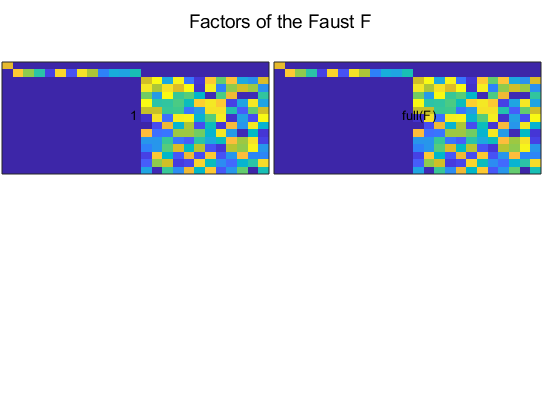This blockdiag projector above is defined in order to keep three blocks of the input matrix A, from the upper-left to the lower-right: the first block is the singleton block composed only of the entry (1,1), the second block is a bit of the next row starting from entry (2,2) and finishing to the entry (2, 13) (its shape is (1,12)) and the final block starts from the element (3,14) to finish on the element (size(R,1), size(R,2)). It's important that the list of blocks covers the whole matrix from its entry (1,1) to its entry (size(R,1), size(R,2)) or the projector will end up on an error. In other words, if you sum together the first (resp. the second) coordinates of all pair of shapes you must find size(R, 1) (resp. size(R, 2)).
The CIRC, TOEPLITZ and HANKEL projectors
These projectors all return the closest corresponding structured matrix (circ being the short name for circulant). For detailed definitions of these kinds of matrices you can refer to wikipedia:
The output is constant along each 'diagonal' (resp. 'anti-diagonal'), where the corresponding constant value is the mean of the values of the input matrix along the same diagonal (resp. anti-diagonal).
In the following example, a Faust is constructed with in first factor a circulant matrix, in second position a toeplitz matrix and at the end a hankel matrix.
import matfaust.proj.*
CI = rand(10, 10);
TI = rand(10, 15);
HI = rand(15, 10);
cp = circ(size(CI));
id = 11
id = 11
tp = toeplitz(size(TI));
id = 10
id = 10
hp = hankel(size(HI));
id = 12
id = 12
F = Faust({cp(CI), tp(TI), hp(HI)});
imagesc(F)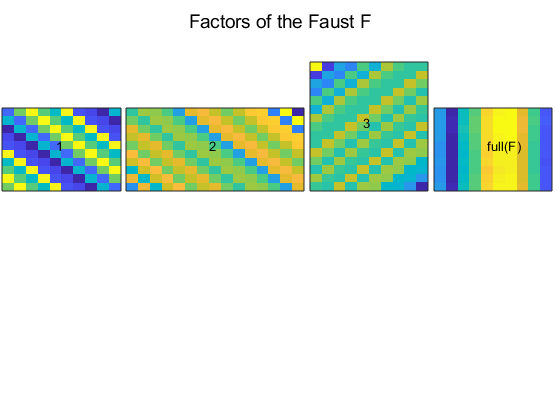You should recognize clearly the structures of a circulant matrix, a toeplitz matrix and a hankel matrix.
Note that these projectors are also capable to receive the normalized and pos keyword arguments we've seen before.
The API documentation for these projectors is available here:
The NORMLIN and NORMCOL projectors
The pyfaust.proj module provides two projectors, normlin (resp. normcol) that project a matrix onto the closest matrix with rows (resp. columns) of a prescribed 2-norm.
Let's try them:
import matfaust.proj.normcol
import matfaust.proj.normlin
pnl = normlin(size(A), 's', .2);
pnc = normcol(size(A), 's', .2);
% let's verify the norm for one column obtained by normlin
P = pnl(A);
norm(P(2,:))
ans = 0.2000
% and the same for normcol
P = pnc(A);
norm(P(:,2))
ans = 0.2000
Something that is important to notice is the particular case of zero columns or rows. When the NORMLIN (resp. NORMCOL) projector encounters a zero row (resp. a zero column) it simply ignores it. Let's try:
B = A;
B(:,2) = 0;
PB = pnc(B);
PB(:,2)
ans = 5×1
0 0 0 0 0
The column 2 is set to zero in B and stays to zero in the projector image matrix.
The SUPP projector
The supp projector projects a matrix onto the closest matrix with a prescribed support. In other words, it preserves the matrix entries lying on this support. The others are set to zero. The support must be defined by a binary matrix (the dtype must be the same as the input matrix though).
import matfaust.proj.supp
% keep only the diagonal of A
ps = supp(eye(size(A))); % by default normalized and pos are false
ps(A)
ans = 5×5
35.7832 0 0 0 0 0 22.3264 0 0 0 0 0 97.5245 0 0 0 0 0 42.4836 0 0 0 0 0 98.6554
This projector is also capable to receive the normalized and pos keyword arguments.
The CONST projector
This last projector is really simple, it returns a constant matrix whatever is the input matrix. The way it's instantiated is very similar to the SUPP projector. Look at its documentation to get an example: const(ant) projector.
Thanks for reading this notebook, you'll find others on the FAµST website. Any feedback is welcome, all contact information is as well available on the website.
Note: this livescript was executed using the following matfaust version:
matfaust.version()
ans = '2.10.32'